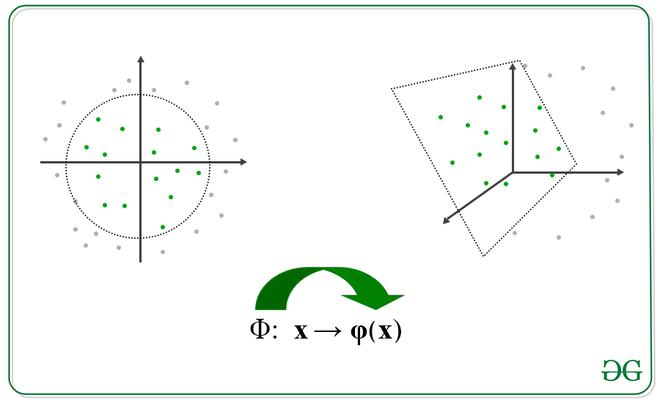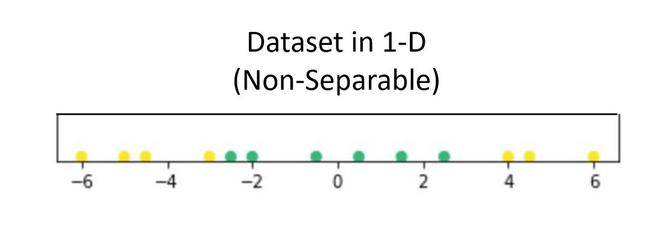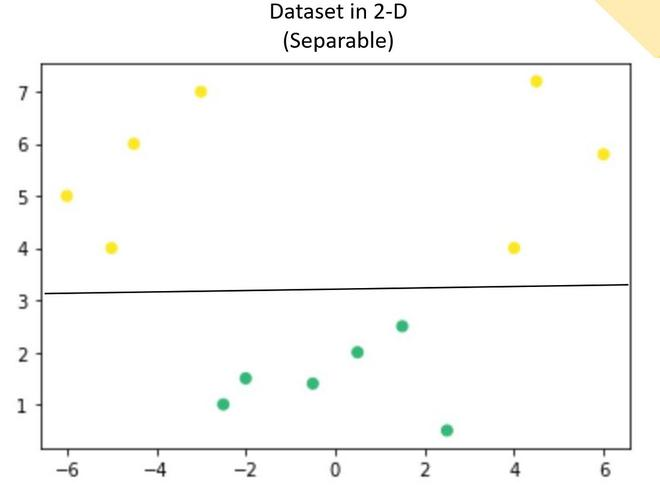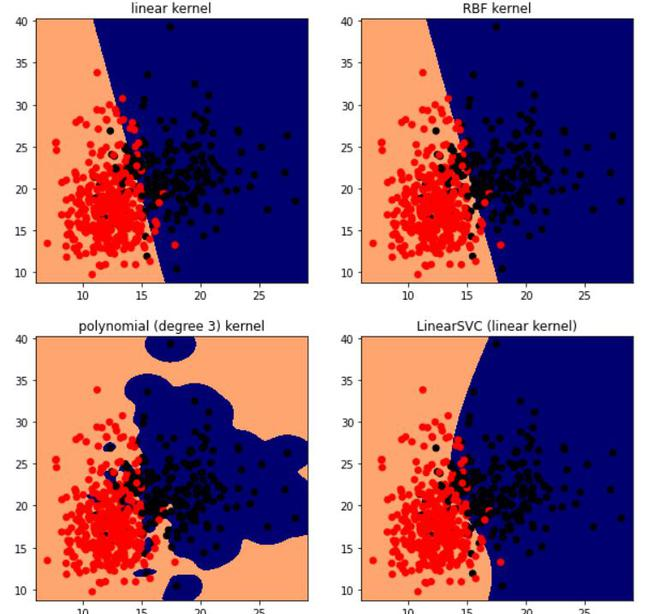# Dual Support Vector Machine

• Last Updated : 30 May, 2021

Pre-requisite: Separating Hyperplanes in SVM

The Lagrange multiplier equation for the support vector machine. The equation of that can be given by:Now, according to the duality principle, the above optimization problem can be viewed as both primal (minimizing over w and b) or dual (maximizing over a).Slater condition for convex optimization guarantees that these two are equal problems.

To obtain minimum wrt w and b, the first-order partial derivative wrt these variables must be 0:Now, put the above equation in the Lagrange multiplier equation and simplify it.In the above equation, the termbecause, b is just a constant and the rest is from the above equation”To find b, we can also use the above equation and constraint:Now, the decision rule can be given by:Notice, we can observe from the above rule that the Lagrange multiplier just depends upon the dot product of xi with unknown variable x. This dot product is defined as the kernel function, and it is represented by KNow, for the linearly inseparable case, the dual equation becomes:Here, we added a constant C, it is required because of the following reasons:

• It prevents the value offrom.
• It also prevents the models from overfitting, meaning that some misclassification can be accepted.Image depicting transformation

we apply transformation into another space such that the following. Note, we don’t need to specifically calculate the transformation function, we just need to find the dot product of those to get kernel function, however, this transformation function can be easily established.where,is the transformation function.

The intuition behind that many of time a data can be separated by a hyperplane in a higher dimension. Let’s look at this in more detail:

Suppose we have a dataset that contains only 1 independent and 1 dependent variable. The plot below represents the data:Now, in the above plot, it is difficult to separate a 1D-hyperplane (point) that clearly separates the data points of different classes. But when this transformed to 2d by using some transformation, it provides options for separating the classes.In the above example, we can see that an SVM line can clearly separate the two classes of the dataset.

There is some famous kernel that is used quite commonly:

• Polynomials with degree =n• Polynomials with degree up to n• Gaussian/ RBF kernel## Python3

 # codeimport numpy as npimport matplotlib.pyplot as pltfrom sklearn import svm, datasets  # import some datacancer = datasets.load_breast_cancer()X = cancer.data[:,:2]Y = cancer.target  X.shape, Y.shape  # perform svm with different kernel, here c is the regularizerh = .02C=100lin_svc = svm.LinearSVC(C=C)svc = svm.SVC(kernel='linear', C=C)rbf_svc = svm.SVC(kernel='rbf', gamma=0.7, C=C)poly_svc = svm.SVC(kernel='poly', degree=3, C=C)  # Fit the training dataset.lin_svc.fit(X, Y)svc.fit(X, Y)rbf_svc.fit(X, Y)poly_svc.fit(X, Y)  # plot the resultsx_min, x_max = X[:, 0].min() - 1, X[:, 0].max() + 1y_min, y_max = X[:, 1].min() - 1, X[:, 1].max() + 1xx, yy = np.meshgrid(np.arange(x_min, x_max, h),np.arange(y_min, y_max, h))  titles = ['linear kernel',          'LinearSVC (linear kernel)',          'RBF kernel',          'polynomial (degree 3) kernel']  plt.figure(figsize=(10,10))  for i, clf in enumerate((svc, lin_svc,rbf_svc, poly_svc )):    # Plot the decision boundary using the above meshgrid we generated    plt.subplot(2, 2, i + 1)    Z = clf.predict(np.c_[xx.ravel(), yy.ravel()])      # Put the result into a color plot    Z = Z.reshape(xx.shape)    plt.set_cmap(plt.cm.flag_r)    plt.contourf(xx, yy, Z)      # Plot also the training points    plt.scatter(X[:, 0], X[:, 1], c=Y)      plt.title(titles[i])  plt.show()

((569, 2), (569,))SVM using different kernels.

### References:

My Personal Notes arrow_drop_up